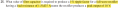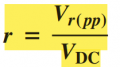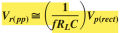# Sizing Filter Capacitor for full wave rectifier

#### SamR

Joined Mar 19, 2019
4,810
I seem to be missing some value needed...Full-wave rectifier (It's a US book so) f = 120Hz assumed
Vout peak = 18V
1% ripple factor = 100%(r) .: r = 0.01
Andbut I don't have either value yet.
ThenNow I can solve that for C but I don't have the ripple Vpp but I do know it has a peak Vout of 18V. So, can I assume from the 1% ripple that it would then be 1% of 18V? .: Vr pp = 18V/100 = 0.18Vpp ripple and the VDC out is 18V - 0.09V= 17.91VDCout.
then C = ?

HINT: the book answer key gives 556 uF and putting into the Vr pp equation gives me a 180uVr pp, hmmm...

Last edited:

#### BobTPH

Joined Jun 5, 2013
7,514
The Vroo in that equation should be 0.18. Then I get 555.56 uF.

Bob

•SamR

#### MrChips

Joined Oct 2, 2009
29,200
C = I / (2f x Vripple)

#### BobTPH

Joined Jun 5, 2013
7,514
I would hope so, since if you substitute V/R for I then it is the same equation.

Bob

#### SamR

Joined Mar 19, 2019
4,810
C = I / (2f x Vripple)
That's a whole lot easier, now if I could only remember it tomorrow...

#### crutschow

Joined Mar 14, 2008
32,848
C = I / (2f x Vripple)
That's assuming the source and rectifiers have negligible resistance.

#### wayneh

Joined Sep 9, 2010
17,405
It's also an approximation. If you actually model it out, the ripple will be a bit less. I get 170mV for 555.6µF. 180mV at 524µF.

Last edited:
•SamR

#### SamR

Joined Mar 19, 2019
4,810

#### wayneh

Joined Sep 9, 2010
17,405
You modeled it in LTS?
Haven't tried that but years ago I set up an Excel spreadsheet to make the precise calculations. There's no analytical expression to give that, as far as I know.
It does give me a bit to think about instead of simply throwing in a 1000uF filtering cap.
Maybe. It is a more-is-better sort of thing.

#### crutschow

Joined Mar 14, 2008
32,848
Maybe. It is a more-is-better sort of thing.
Not always.

The downside of a larger than needed filter capacitor, is that the peak RMS current is higher through the transformer windings, giving more I²R loss and heat in the transformer.
That high peak current is the reason a transformer current rating should be derated nearly 50% for a diode-capacitor DC supply current value.

•SamR

#### MrAl

Joined Jun 17, 2014
10,587
Hi,

There is an exact solution to this problem but most people dont want to be bothered with it. It's very interesting though. You have to solve for both the voltage (and current) and time. You can then calculate the peak to peak ripple and average DC voltage.

It might be more interesting for folks here to note that the average DC voltage changes with different capacitor values. This occurs because the energy stored in the cap for each half cycle increases with larger capacitor values. Simulations will show this effect.

•SamR

#### SamR

Joined Mar 19, 2019
4,810
Yes, but I'm working with a non-calculus book... I haven't used calculus since college so I'll settle for the derivatives.

•MrAl

#### MrAl

Joined Jun 17, 2014
10,587
Yes, but I'm working with a non-calculus book... I haven't used calculus since college so I'll settle for the derivatives.
Yes much easier unless you are interested in theory partly for the sake of theory•SamR

#### wayneh

Joined Sep 9, 2010
17,405
There is an exact solution to this problem...
By "exact" do you mean an analytical solution? If so I'd love to see it but I doubt that it's possible.

My spreadsheet does the exact calculation from an engineering standpoint but a mathematician would faint over using that language. I mean it's iterative to any level of precision Excel is capable of. Might as well be a mile to a mathematician.

#### michael8

Joined Jan 11, 2015
355
After the math you are going to pick an electrolytic capacitor with a tolerance of +/- 20% or perhaps -10% +100%....

C = I / (2f x Vripple)
That's a whole lot easier, now if I could only remember it tomorrow.

An approximation is easy:

the voltage change of a capacitor is Q/C where Q is the change in charge and C is the capacitance

current is charge * time or Q*t

t = time in seconds

the time is when the input is absent and the load is just ramping the capacitor voltage down, this happens twice
a cycle so time for 60 Hz is (1/60)/2 -> .0083 seconds (8.3 mS)

so the change in voltage on the capacitor is V = (I * t) / C

your voltage change is .18 volts

and the current is approximately 18/1500 -> .012 Amps (12mA)

rearrange the voltage on a capactor equation

C = (I * t) / V -> (.012 * .0083) / .18 -> .000553333 (or 553uF)

#### MrAl

Joined Jun 17, 2014
10,587
By "exact" do you mean an analytical solution? If so I'd love to see it but I doubt that it's possible.

My spreadsheet does the exact calculation from an engineering standpoint but a mathematician would faint over using that language. I mean it's iterative to any level of precision Excel is capable of. Might as well be a mile to a mathematician.
Hello there,

Well i am not the only one to think about this i just wanted to come up with a solution that is so general that it could be used for any rectifier circuit.
A professor from some university did something like this but he only did one particular circuit.
I will post the equation but you may not like it :=)
The solution is analytical for some circuits but is only semi analytical for others. I use the phrase "semi analytical" because it is NOT a simulation it is analytical over one half cycle (or one full cycle perhaps) meaning that the calculation comes out exact for one half cycle, then for another half cycle, etc. So there is never a time when we make a 'step' like 1 microsecond, it's always much much larger than that and not an approximation like a simulation is.

It is interesting too though that for some of the simpler circuit a complete analytical solution is possible but we still need to use a numerical solver. That solution however is complete for that entire half cycle not just 1us or something, and in theory it is exact because we can use a numerical solver of any precision. If we did this in a simulator type environment it would take forever to solve for the same half cycle.

i'll find the solution again. I had posted it here several times already but in thread fairly old by now.

Ok here it is. Note that this says "with cap ESR" but that Equ 1 is for any circuit that's the beauty of it.
What helps in finding the particular solutoin(s) is to apply 'continuity of states' which allows a half cycle by half cycle solution rather than a microsecond by microsecond solution. So the math covers an entire half cycle without actually having to calculate every point.
I also have the professors solution and that is pretty good but does not include cap ESR. I can post that too you might like that better it's all algebra and trig.

Last edited: